Chin. Phys. B, 2020, Vol. 29(10): 108201    DOI: 10.1088/1674-1056/abaedc
 INTERDISCIPLINARY PHYSICS AND RELATED AREAS OF SCIENCE AND TECHNOLOGY Prev   Next

# Distribution of a polymer chain between two interconnected spherical cavities

Chao Wang(王超)1,†(), Ying-Cai Chen(陈英才)1, Shuang Zhang(张爽)2, Hang-Kai Qi(齐航凯)3, Meng-Bo Luo(罗孟波)3,‡()
1 Department of Physics, Taizhou University, Taizhou 318000, China
2 College of Science, Beibu Gulf University, Qinzhou 535011, China
3 Department of Physics, Zhejiang University, Hangzhou 310027, China
Abstract

The equilibrium distribution of a polymer chain between two interconnected spherical cavities (a small one with radius Rs and a large one with radius Rl) is studied by using Monte Carlo simulation. A conformational transition from a double-cavity-occupation (DCO) state to a single-cavity-occupation (SCO) state is observed. The dependence of the critical radius of the small cavity (RsC) where the transition occurs on Rl and the polymer length N can be described by ${R}_{{\rm{sC}}}\propto {N}^{1/3}{R}_{{\rm{l}}}^{1-1/3\nu }$ with ν being the Flory exponent, and meanwhile the equilibrium number (ms) of monomers in the small cavity for the DCO phase can be expressed as ms = N/((Rl/Rs)3 + 1), which can be quantitatively understood by using the blob picture. Moreover, in the SCO phase, the polymer is found to prefer staying in the large cavity.

Keywords:  polymer      phase transition      free energy      blob theory
Received:  06 July 2020      Revised:  29 July 2020      Published:  05 October 2020
 PACS: 82.35.Jk (Copolymers, phase transitions, structure) 82.35.Lr (Physical properties of polymers) 82.20.Wt (Computational modeling; simulation)
Corresponding Authors:  Chao Wang(王超), Meng-Bo Luo(罗孟波)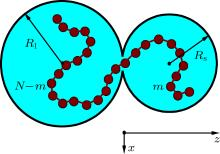Fig. 1.  A 2D sketch of the simulation model. Two spherical cavities, a small one with radius Rs and a large one with radius Rl, are connected by a small hole with diameter Dh. The polymer is confined in the two cavities.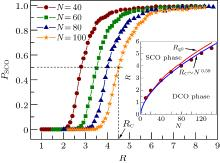Fig. 2.  The SCO probability PSCO as a function of R for different N. The radius where PSCO = 0.5 is defined as the critical radius RC. The inset shows the phase diagram for the symmetric twin-cavity system. The red line shows the radius of gyration (Rg0) of polymer in free space as a function of N.Fig. 3.  The SCO probability PSCO as a function of the radius of the small cavity Rs for different Rl (< RC), where N = 60. The radius where PSCO = 0.5 is defined as the critical radius RsC.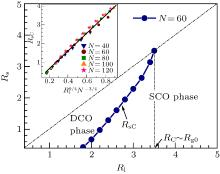Fig. 4.  Phase diagram for the asymmetric system, where N = 60. The inset shows the dependence of RsC on ${R}_{{\rm{l}}}^{9/4}{N}^{-3/4}$ for N = 40, 60, 80, 100, and 120. The solid black line is guide for eyes.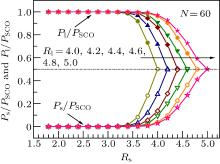Fig. 5.  The relative probabilities of the whole polymer in the small cavity (Ps/PSCO) and in the large cavity (Pl/PSCO) in the SCO phase as functions of the radius of the small cavity Rs for different Rl, where N = 60.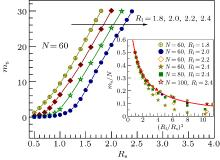Fig. 6.  The equilibrium number (ms) of monomers in the small cavity as a function of Rs for different Rl (< RC), where N = 60. The inset shows ms/N as a function of (Rl/Rs)3 for different Rl and N. The solid red line is given by Eq. (12).